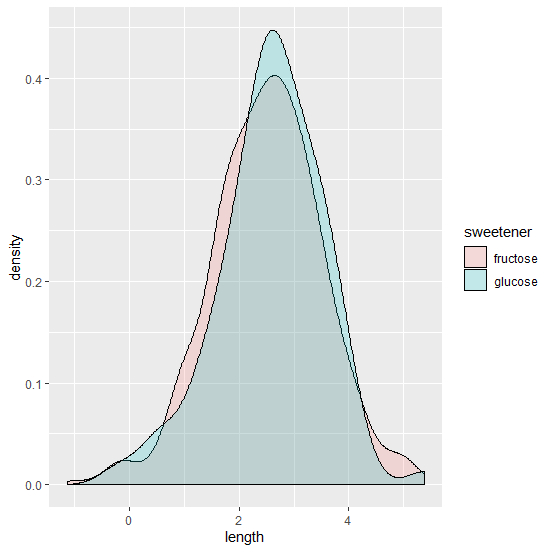# How to plot two histograms together in R?

Consider the below data frames −

> glucose  <- data.frame(length = rnorm(100, 2.5))
> fructose <- data.frame(length = rnorm(500, 2.5))

We need to combine these two data frames but before that we have to make a new column in each of these data frames to create their identification

> glucose$sweetener <- 'glucose' > fructose$sweetener <- 'fructose'
> sweeteners <- rbind(glucose, fructose)

Now let’s create the histograms

> library(ggplot2)
> ggplot(sweeteners, aes(length, fill = sweetener)) + geom_density(alpha = 0.2)